﻿ Numerical Aerodynamic of the Rectangular Wing Concerning to Ground EffectPublications are Open
Access in this journal
Article Versions
Export Article
• Normal Style
• MLA Style
• APA Style
• Chicago Style
Research Article
Open Access Peer-reviewed

### Numerical Aerodynamic of the Rectangular Wing Concerning to Ground Effect

Alireza Heidarian, Hassan Ghassemi , Pengfei Liu
American Journal of Mechanical Engineering. 2018, 6(2), 43-47. DOI: 10.12691/ajme-6-2-1
Published online: April 21, 2018

### Abstract

Numerical aerodynamic of the rectangular wing is presented regarding to the ground effect. Clark-Y wing section is selected for the present calculations. The Reynolds-averaged Navier-Stokes (RANS) equations solver of the ANSYS-CFX software is employed with a realizable k-ε turbulent model. The numerical results lift and drag of the rectangular airfoil at ground effect are verified with the experimental data. Pressure distribution and velocity contour and turbulent intensity at the trailing edge of the airfoil are presented and discussed.

### 1. Introduction

It is obvious that several countries are working on the development of wing-in-ground-effect (WIG) craft for different purposes such as carrying traveller and cargo, salvage and rescue, and military commissions. When a wing is placed in proximity to the ground, lift force rises and drag force decreases and consequently, lift-to-drag ratio improves; in another word, the efficiency of wing increases 1.

Wing and foil are lofting bodies that mean lift-drag ratio is high. Lift is generated by pressure, calculations of the pressure is an essential on those bodies. There are many numerical methods to determine the pressure force and frictional force. Hydrofoil is operating beneadth the free surface and generates the wave. Hydrofoil and wing are similar each other. When wing is operating above the ground is similar to the hydrofoil is under the free surface. Ghassemi et al. 2, 3, 4 employed the boundary element method (BEM) to the hydrofoil at various conditions affected by the free surface. Performance predicting of 2D and 3D submerged hydrofoils using CFD and ANNs carried out by Nowruzi et al 5.

The flow structure under the suction surface of an inverted wing is constrained with ground level. The flow stimulates considerably as the wing approaches the ground, which results in higher suction and consequently greater downforce as compared with free stream 6. Driss et al. experimentally studied a thick trailing edge of wing by means of Laser Doppler Anemometry (LDA). They showed two counter–rotating vortices in the trailing edge and analyzed the downstream flow 7. Pavelka and et al experimentally studied the turbulent wake of flow behind the trailing edge of an inverted airfoil in proximity to the ground. They showed that the wake region developed as the height of the wing decreased 8. Jia and et.al experimentally explored the wake region at the downstream of an inverted wing. They showed that the size of the wake increased but the velocity deficit reduced while moving - downstream. An adverse pressure gradient of flow was observed between the wake region and the ground surface 9. There are some methods to control flow separation, for instance, vortex generators (VGs) and vortex generator jets. Vortex generator is a method to decrease the drag on the foil and wing, so it is numerically studied by Ashrafi et al 10.

Zerihan and Zhang numerically analyzed the pressure distribution and wake region around a single element. Reynolds-averaged Navier–Stokes (RANS) method was exercised for 2D simulation with a structured grid, and the SS, k-w and Saplart-Allmaras turbulence models were employed for turbulent flow around the wing 11. Aerodynamics of a airfoil in dynamic ground effect have numerically studied by Qu et al 12, 13.

In the present work, the physics of flow around a rectangular wing in ground effect was investigated. The pressure distribution around the rectangular wing, as well as velocity and turbulent intensity distributions in the wake region behind the wing were examined. This study was performed at different angles of attack in ground effect. This work showed an in-depth investigation of flow structure around and behind a rectangular wing, which researchers and designers can eventually use the outcome as a guideline for wing in ground effect.

### 2. Governing Equations

The turbulent flow around the wings was introduced as steady–state and incompressible using realizable k-ε turbulent model. The ANSYS CFX software was applied for computational fluids dynamics (CFD) simulations. The transport equations for the turbulent kinetic energy (k) and turbulent dissipation energy (ε) were proved as follows: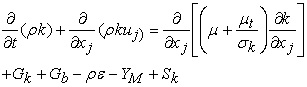(1)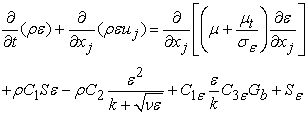(2)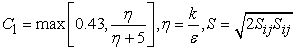(3)

where Sk and Sε are user-defined source terms, while C, C2, C, σk and σε are the adaptable constants. The pressure coefficient (CP), aerodynamic lift and drag coefficients (CL, CD) are defined as follows: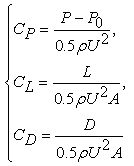(4)

where A is the platform area, L and D are lift and drag, respectively. P0 is atmosphere pressure and P is dynamic pressure at wing surface. U and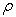are free stream velocity and water density, respectively.

### 3. Results and Discussions

The computational analysis was implemented using a common rectangular Clark-Y airfoil section. The major dimensions of the rectangular wing (Figure 1). Numerical calculations were performed using the commercial computational fluid dynamics code ANSYS CFX 14.5 14. RANS was used in our simulations using steady-state with the realizable k-ε turbulence model.

The wing has span 25 cm with 20 cm in chord. Aspect ratio is 1.25 that is low value. When the aspect ratio is low, induced drag is high and cross flow may effect on the stronger tip vortex. Therefore, aerodynamic analysis is much important when it is near the ground. These simulations were prepared with respect to various angles of attack and ground clearance (h/c) of 0.15 with the aspect ratio of 1.25. The air velocity in the inlet was 25.5 m/s. The ground was assumed to move the same speed as air velocity. Ground level (h) is defined as the distance between the trailing edge of centre wings and ground surface.

• Figure 1. The rectangular wing

A symmetry plane was employed for the rectangular wing to reduce usage memory and time consumption. These simulations were designed with a consideration an appropriate physical computational domain that was deemed to have no blockage effects from the side and top. In addition, there was enough space between wing surface and the inlet and outlet boundaries to avoid interactions among them.

The CFD simulation was validated with experimental results using the low-speed wind tunnel at University Technology of Malaysia. This wind tunnel is not equipped by moving ground. Figure 2 (a-b) exhibits a comparison between numerical and experimental drag coefficients and lift-to-drag ratios of the rectangular wing for fixed ground. The aerodynamic coefficients for the aspect ratio of 1.25 from the experimental data were introduced. The truth of numerical aerodynamic coefficients was then compared to the experimental results. For validation purposes, the numerical results of a rectangular wing with Clark-Y airfoil section were determined.

The numerical outcomes and experimental data of drag coefficients (CD) are given in Figure 2. The trend of both data had a good contract, but the computational results were insignificantly larger at greater angles. The increase of drag coefficient due to the increment of the angle of the attack explained the same trend in numerical and experimental simulations.

The variation of pressure coefficient (Cp) at the middle span on the surface of the rectangular wing was studied at low ground clearance of 0.15c for different angles of attack of 4° and 6° as shown in Figure 3. The positive pressure on the lower surface and negative pressure (suction effect) on the upper surface of the wing increased, hence the angle of attack augmented. At the angle of attack of 4°, the pressure on the lower side nearby the leading edge reached negative value, while all pressure was positive on the lower surface at the angle of attack of 6°. Adverse pressure gradients are shown (Figure 3) to be slightly higher on the upper surface for 6°, which produced lower momentum and subsequently the velocity defect was greater in the wake region as revealed in Figure 4.

The tendency of the aerodynamic coefficients showed a good agreement between computational and experimental results, though the numerical data had some discrepancies from experimental measurement.

The present investigation studied the physics of flow around and behind a rectangular wing. In the study, the pressure distribution around wings and the wake region behind trailing edges were illustrated at low ground clearance of 0.15*chord length (0.15C) and two angles of attack of 4° and 6°.

• Figure 2. Comparison of experimental and numerical simulation of the rectangular wing at ground clearance of 0.15: (a) Drag coefficient; (b) Lift-to-drag ratio
• Figure 3. Pressure coefficient (Cp) at the middle span of the rectangular wing at h/c= 0.15 at two angles of attack (α=4, 6 deg)
• Figure 4. Velocity contours (m/s) at the middle span of the rectangular wing at h/c= 0.15 at two angles of attack (α=4, 6 deg)
• Figure 5. Turbulent intensity at the trailing edge of the rectangular wing at h/c= 0.15 at two angles of attack (α=4, 6 deg)

Figure 4 illustrates the velocity distribution around and behind the rectangular wing. There were higher velocity distributions at the middle span of the rectangular wing between the lower surface and the moving ground at smaller angle of attack (according to Bernoulli equation, the pressure should be higher for higher angle of attack, i.e. α=6°). The movement of stagnate air flow from leading of the wing towards the lower surface was greater for higher angle of attack that caused higher ram pressure. Conversely, some parts of the air flow that distracted the upper surface had lower momentum, and then the suction effect was weaker for higher angle of attack as compared with lower angle of attack. Based on the velocity contours, the probability for separation of airflow for the higher angle of attack was a slightly greater due to the lower kinetic energy of the air flow on the upper surface of the wing. The velocity contours in the wake region behind the rectangular wing showed that velocity defect had an augmentation, therefore the angle of attack increased at low ground clearance of 0.15. Figure 5 illustrates the turbulent intensity contours at the trailing edge and behind he rectangular wing in ground effect. When the angle of attack was augmented, the turbulence level of air flow in the district of the trailing edge showed a slight improvement.

The mean velocity and turbulence intensity distributions were achieved in the wake region behind the rectangular wing at various axial directions (x/c= 1.05, 1.25, 1.5, 2, 2.5 and 3) from the ground until y/c= 1.15. The reference for x and y coordinates is the leading edge and the ground surface respectively. This comparison indicates the maximum defect velocity, thickness of the wake region and the peak of turbulent intensity. The maximum velocity defect in the wake region behind the rectangular wing was determined as 44% and 46% at the angles of attack of 4° and 6° respectively

### 4. Conclusions

The present study numerically carried out the pressure and velocity distribution, lift and drag on the rectangular airfoil. At higher angle of attack, positive pressure on the lower surface suction effect was documented on the upper surface of the wing, which was stronger than that of the lower angle. The adverse pressure gradient was slightly higher on the upper surface for higher angle of attack. The angle of attack has slight effect on velocity distribution and turbulence level in the wake region. The velocity defect and turbulent intensity near the trailing edge of the wing for higher angle of attack were predicted to be greater than that of the lower angle, but these differences reduced as the flow moved downstream. The velocity defect and turbulent intensity were approximately two times of the chordwise from the trailing edge in the wake region. The position of the maximum velocity defect and turbulence level was around the ground clearance of the trailing edge of the airfoil, and this position shifted to ground with the axial distance from the trailing edge.

### References

  Jamei, S., Maimum A., Mansour S., Azwadi, N., Priyanto A., Numerical Investigation on Aerodynamic Characteristics of a Compound Wing-in-Ground Effect. Journal of Aircraft, 2012. 49(5): p. 1297-1305. In article View Article  Ghassemi H., Kohansal AR., Ardeshir A., Higher order boundary element method applied to the hydrofoil beneath the free surface, ASME 2009 28th International Conference on Ocean, Offshore and Arctic Eng. 2009, 597-602. In article  Ghassemi H, Kohansal AR., Isoparametric boundary element method applied to the rectangular and delta hydrofoils near the free surface, China ocean engineering, 2010, 24 (2), 321-331. In article  Ghassemi H., Kohansal AR., Wave generated by the NACA4412 hydrofoil near free surface, Journal of Applied Fluid Mechanics (JAFM), 2013, 6 (1), 1-6. In article  Nowruzi H., Ghassemi H., Ghiasi M., Performance predicting of 2D and 3D submerged hydrofoils using CFD and ANNs, Journal of Marine Science and Technology, 2017, 22 (4), 710-733. In article View Article  Tofa, M.M., et al., Experimental Investigation of a Wing-in-Ground Effect Craft. The Scientific World Journal, 2014. 2014: p. 7. In article  Driss, S., Z. Driss, and I.K. Kammoun, Computer Simulation of the Aerodynamic Structure of an Arched Roof Obstacle and Validation with Anterior Results. American Journal of Mechanical Engineering, 2015. 3(3A): p. 9-14. In article  Pavelka, P., R.b. Hu¨¾ady, and M. Hagara, Result Correlation of Experimental and Numerical Modal Analysis of a Steel Beam. American Journal of Mechanical Engineering, 2016. 4(7): . 376-379. In article  Jia, Q., W. Yang, and Z. Yang, Numerical study on aerodynamics of banked wing in ground effect. International Journal of Naval Architecture and Ocean Engineering, 2016. 8(2): p. 209-217. In article View Article  Ashrafi A., Ketabdari M., Ghassemi H., Numerical Study on Improvement of Hydrofoil Performance Using Vortex Generators (Research Note), International Journal of Engineering-Transactions B: Applications, 2014, 28 (2). In article  Zerihan, J. and X. Zhang, Aerodynamics of a Single Element Wing in Ground Effect. Journal of Aircraft, 2000. 37(6): p. 1058-1064. In article View Article  Qu, Q., Jia X., Wang W., Liu P., Agarwal R.K., Numerical study of the aerodynamics of a NACA4412 airfoil in dynamic ground effect. Aerospace Science and Technology, 2014. 38: p. 56-63. In article View Article  Qu, Q., Lu Z., Liu P., Agarwal R.K. Numerical Study of Aerodynamics of a Wing-in-Ground-Effect Craft. Journal of Aircraft, 2014. 51(3): p. 913-924. In article View Article  ANSYS CFX-Solver Theory Guide. 2011. In article

Published with license by Science and Education Publishing, Copyright © 2018 Alireza Heidarian, Hassan Ghassemi and Pengfei LiuThis work is licensed under a Creative Commons Attribution 4.0 International License. To view a copy of this license, visit http://creativecommons.org/licenses/by/4.0/